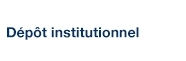# On the algebraic components of the SL(2,C) character varieties of knot exteriors

Boyer, Steven; Luft, E. et Zhang, X. (2002). « On the algebraic components of the SL(2,C) character varieties of knot exteriors ». Topology, 41(4), pp. 667-694.

Fichier(s) associé(s) à ce document :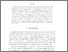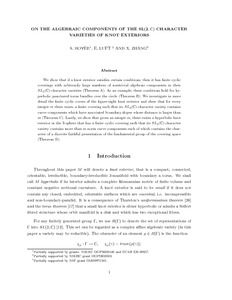Prévisualisation
PDF
Télécharger (300kB)

## Résumé

We show that if a knot exterior satisfies certain conditions, then it has finite cyclic coverings with arbitrarily large numbers of nontrivial algebraic components in their SL(2,Full-size image (<1 K)) character varieties (Theorem A). As an example, these conditions hold for hyperbolic punctured torus bundles over the circle (Theorem B). We investigate in more detail the finite cyclic covers of the figure-eight knot exterior and show that for every integer m there exists a finite covering such that its SL(2,Full-size image (<1 K)) character variety contains curve components which have associated boundary slopes whose distance is larger than m (Theorem C). Lastly, we show that given an integer m then there exists a hyperbolic knot exterior in the 3-shpere which has a finite cyclic covering such that its SL(2,Full-size image (<1 K)) character variety contains more than m norm curve components each of which contains the character of a discrete faithful presentation of the fundamental group of the covering space (Theorem D).

Type: Article de revue scientifique hyperbolic knot Faculté des sciences > Département de mathématiques Steven P. Boyer 27 avr. 2016 13:39 19 mai 2016 18:00 http://archipel.uqam.ca/id/eprint/8324

## Statistiques

Voir les statistiques sur cinq ans...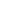RECHERCHER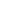PARCOURIR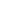LIBRE ACCÈS